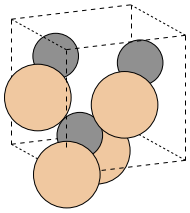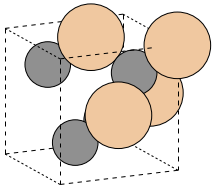Structure optimization¶

This tutorial is concerned with structural optimization. Except for high-symmetry structures - like the cubic crystal we are using in this tutorial the determination of the correct structure for the investigated system is a crucial part in the workflow of the computation. The Quantum-Espresso package is able to do a full structural optimization of the crystal and the QE-util provides an interface to this mode of operation. To continue skip over the initial setup to the second cell below.

In :
# Import the basic libraries

# ASE system
import ase
from ase import Atom, Atoms
from ase import io
from ase.lattice.spacegroup import crystal
from ase.units import GPa, Bohr, Rydberg

# Spacegroup/symmetry library
from pyspglib import spglib

# iPython utility function
from IPython.core.display import Image

# Import the remote execution tools from the qe-util package
from qeutil import RemoteQE

# Access info
import host

qe=RemoteQE(label='SiC-structure',   # A name for the project
kpts=[3,3,3],    # k-space grid
xc='pz',         # Exchange functional type in the name of the pseudopotentials
pp_type='vbc',   # Variant of the pseudopotential
pp_format='UPF', # Format of the pseudopotential files
ecutwfc=70,      # Energy cut-off
pseudo_dir='../pspot',
use_symmetry=True,
procs=4)         # Use 8 cores for the calculation

print qe.directory
calc/SiC-structure.GHl7tH

Preparations¶

To start any calculation we need to create a crystal. We will again use a cubic, zinc-blende (F-43m) SiC crystal again with a lattice constant determined in our first tutorial. But this time we will distort it randomly to have a low-symmetry structure with many potential degrees of freedom and configuration displaced from the equilibrium position. Our task is to find the equilibrium structure - which hepefully will be a high-symmetry cubic crystal with a F-43m space group symmetry.

In :
a=4.344
cryst = crystal(['Si', 'C'],
[(0, 0, 0), (0.25, 0.25, 0.25)],
spacegroup=216,
cellpar=[a, a, a, 90, 90, 90])

# Assign the calculator to our system
cryst.set_calculator(qe)

# Verify the symmetry
print "Symmetry group:", spglib.get_spacegroup(cryst)
Symmetry group: F-43m      (216)
In :
print "Stress tensor  (Voigt notation, GPa):\n", cryst.get_stress()/GPa
Stress tensor  (Voigt notation, GPa):
[ 0.054  0.054  0.054  0.    -0.     0.   ]

Let us distort randomly the positions of atoms in the unit cell as well as the unit cell itself. We will use standard deviation of 0.05 (5% of the unit cel size). This should generate a completely non-symmetric crystal (group P1).

In :
cryst.rattle(stdev=0.05)
cryst.set_cell(diag(1+0.01*randn(3))*cryst.get_cell(), scale_atoms=True)
In :
# Verify that indeed we have a low symmetry structure
print "Symmetry group:", spglib.get_spacegroup(cryst)
Symmetry group: P1         (1)
In :
# Display the structure
ase.io.write('crystal.png', cryst, format='png', show_unit_cell=2, rotation='115y,15x', scale=30)
Image(filename='crystal.png')
Out:Structure check¶

Let us check the effect the distorsion has on the crystal by calculating the stress tensor and forces acting on atoms in the distorted structure. Note that both forces as well as stresses are far from zero.

In :
print "Stress tensor  (Voigt notation, GPa):\n", cryst.get_stress()/GPa

# Print also the forces (eV/A)

print "\nForces on atoms (eV/A)"
print "======================"
print cryst.get_forces()
Stress tensor  (Voigt notation, GPa):
[-1.445 -1.858  2.36  -4.608 -3.146 -1.636]

Forces on atoms (eV/A)
======================
[[-0.00182192 -0.00303699 -0.00235983]
[-0.00233322 -0.00147804 -0.00041353]
[-0.00242831 -0.0023165   0.00020909]
[-0.00177738  0.00091272 -0.00011439]
[ 0.00153439  0.00353868  0.00321501]
[ 0.00208721  0.00029438 -0.0003175 ]
[ 0.00309936  0.00178758 -0.00212825]
[ 0.00163988  0.00029818  0.00190939]]

Optimization procedure¶

Our goal is to find the the structure as close as possible to minimum of energy (i.e. with as small as possible stresses on the unit cell and forces on atoms). The Quantum Espresso has a special mode of working where it minimizes forces and stresses. The QE-util package provides full access to this mode by the way of calc parameter of the calculator object. There are two variants of the optimization procedure:

• 'relax' changes just the atomic positions keeping the cell fixed
• 'vc-relax' which changes atomic positions as well as unit cell shape and size

We will first try to optimize the internal degrees of freedom (atomic positions) and in the second step optimize both atomic positions and the unit cell parameters. This is actually a recommended procedure in the case of complicated structures. Running just vc-relax optimization may not converge at all in such cases or, even worse, give you a false minimum.

Note: The optimization runs take much longer then the simple energy calculations. Be patient.

In :
# Switch to the atomic position relaxation mode
qe.set(calc='relax')

# Switch off the use of symmetries.
qe.set(use_symmetry=False)

# Force recalculation by clearing the results from the previous calculation.
qe.reset()
In :
# Run the calculation and get the stresses and forces at the end.
# The structure in cryst is *not* modified
print "Stress:\n", cryst.get_stress()/GPa
print "\nForces:\n", cryst.get_forces()
Stress:
[ 0.276 -0.392  4.008 -0.027 -0.018 -0.012]

Forces:
[[ -2.02115509e-05  -2.47100075e-05  -1.84702755e-05]
[ -3.78708907e-06   2.69222867e-06  -1.12200827e-05]
[ -1.30597594e-05  -6.89003624e-06   4.93017778e-06]
[ -1.27279953e-05  -4.46656372e-06   2.32351547e-06]
[  3.25844458e-05   9.91480688e-06   4.82866497e-06]
[  2.21889115e-06   1.71758900e-05  -4.63847430e-06]
[ -5.96358599e-06  -1.83975441e-05   1.44568246e-05]
[  2.09470326e-05   2.46804482e-05   7.79042766e-06]]

After running the first cycle, we note that the stresses have been reduced mostly to the diagonal part of the tensor (first three numbers in the Voigt notation) and forces have been diminished to the level of $10^{5}$ eV/A. We can improve this result by lowering the convergence condition to the $10^{-8}$ eV/A (note the translation of units to the units required by Quantum Espresso). We change the condition and re-run the calculation on the same structure.

In :
qe.set(forc_conv_thr=1e-8*Rydberg/Bohr)
qe.reset()
In :
print "Stress:\n", cryst.get_stress()/GPa
print "\nForces:\n", cryst.get_forces()
Stress:
[ 0.276 -0.392  4.008 -0.    -0.     0.   ]

Forces:
[[  2.11582259e-07  -5.12620252e-07  -3.57045062e-07]
[ -2.83535784e-07  -2.86258350e-07  -9.60676801e-08]
[ -3.31764094e-07   4.87728222e-07   8.94557345e-08]
[  2.74979149e-07   2.48142429e-07   3.50433116e-07]
[  5.40623787e-07   5.09508749e-08  -1.34572540e-07]
[ -9.15171058e-07   5.61237499e-07   1.48963245e-07]
[ -4.84616718e-07  -4.15385758e-07   6.10632622e-08]
[  9.87513521e-07  -1.33794664e-07  -6.18411382e-08]]

Updating the structure¶

We can see that the forces dropped by another two orders of magnitude and the stress got concentrated even more on the diagonal elements. Thus, let us update the positions in the cryst object with a our new positions. The found positions are present in the results dictionary of the calculator object. We can check the symmetry of the resulting crystal - but it will probably be different from the desired F-43m. Which should be expected - the unit cell is still the same deformed unit cell we have generated at the start.

In :
# Update the positions using calculated values
cryst.set_scaled_positions(qe.results['atomic_positions'])

# Check the symmetry. Probably not the F-43m !
print "Symmetry group:", spglib.get_spacegroup(cryst,symprec=1e-4)
Symmetry group: P1         (1)

Full cell optimization¶

We have approched optimal atomic positions in the unit cell. It is time to search for the optimal shape and size of the unit cell itself. We need to switch to the vc-relax mode and run the optimization again. Then update the structure again - this time both unit cell and atomic positions and check the symmetry.

In :
qe.set(calc='vc-relax')
qe.reset()
In :
print "Stress:\n", cryst.get_stress()/GPa
print "\nForces:\n", cryst.get_forces()
Stress:
[-0.001 -0.001 -0.001 -0.    -0.     0.   ]

Forces:
[[  2.37252165e-08  -1.78911469e-08  -1.78911469e-08]
[ -2.41141545e-08  -7.77875952e-09   2.06137127e-08]
[ -3.50044178e-08   7.77875952e-10   2.95592862e-08]
[  1.16681393e-08   1.94468988e-08   3.22818520e-08]
[ -1.88634918e-07   1.07735819e-07  -3.01037993e-07]
[  1.92913236e-07  -9.17893623e-08  -3.16984450e-07]
[  1.73466337e-07   9.02336104e-08   2.69922955e-07]
[ -1.54019438e-07  -1.01123874e-07   2.83535784e-07]]
In :
# Update the crystal
cryst.set_cell(qe.results['cell'])
cryst.set_scaled_positions(qe.results['atomic_positions'])

# Check the symmetry
print "Symmetry group:", spglib.get_spacegroup(cryst,symprec=1e-4)
Symmetry group: F-43m      (216)

Finishing¶

After a single run the forces/stresses as well as the symmetry will not be converged well enough. You will need to repeat the cycle of updating the crystal and optimizing it again (two cells above) one or two more times - until you reach the appropriate level of forces/stresses and symmetry.

We can conclude the run by "snapping" the structure to the high-symmetry values by rounding the positions and sizes to appropriate number of decimal places.

Finally we check the symmetry of the structure and verify the stresses and forces by running a single point (scf - Self Consistent Field) calculation on the final structure - which should result in stress components below 0.01 GPa and forces below $10^{-6}$ eV/A as well as recovery of the expected F-43m symmetry group of the crystal.

In :
# Round the sizes and positions to get to the high-symmetry structure
cryst.set_cell(np.round(qe.results['cell'],4))
cryst.set_scaled_positions(np.round(qe.results['atomic_positions'],3))

# See the structure
print "Unit cell:\n", cryst.get_cell()
print "\nAtomic positions:\n", cryst.get_scaled_positions()
Unit cell:
[[ 4.3342 -0.     -0.    ]
[-0.      4.3342  0.    ]
[-0.      0.      4.3342]]

Atomic positions:
[[ 0.004  0.994  0.997]
[ 0.004  0.494  0.497]
[ 0.504  0.994  0.497]
[ 0.504  0.494  0.997]
[ 0.254  0.244  0.247]
[ 0.754  0.744  0.247]
[ 0.754  0.244  0.747]
[ 0.254  0.744  0.747]]
In :
# Vierify the symmetry
print "Symmetry group:", spglib.get_spacegroup(cryst)
Symmetry group: F-43m      (216)
In :
# Switch to the single point energy calculation mode
qe.set(calc='scf')
qe.reset()
In :
# Verify the final stresses and forces

print "Stress tensor  (Voigt notation, GPa):\n", cryst.get_stress()/GPa

# Print also the forces (eV/A)

print "\nForces on atoms (eV/A)"
print "======================"
print cryst.get_forces()
Stress tensor  (Voigt notation, GPa):
[-0.024 -0.024 -0.024  0.     0.     0.   ]

Forces on atoms (eV/A)
======================
[[  3.50044178e-09  -8.80944516e-07   6.17633506e-07]
[  2.48920305e-08   8.67720624e-07  -6.30857397e-07]
[  5.79517584e-08  -7.93433471e-07  -5.72127763e-07]
[  7.38982154e-09   8.83278143e-07   6.89975969e-07]
[ -5.62404313e-07  -1.03457502e-06   1.72299523e-07]
[  2.12749073e-07   1.23021082e-06   8.94557345e-08]
[  5.25844144e-07  -1.72688461e-06  -2.76534901e-07]
[ -2.69534017e-07   1.45501697e-06  -8.98446725e-08]]
In :
# Display the structure
ase.io.write('crystal.png', cryst, format='png', show_unit_cell=2, rotation='115y,15x', scale=30)
Image(filename='crystal.png')
Out:In [ ]: